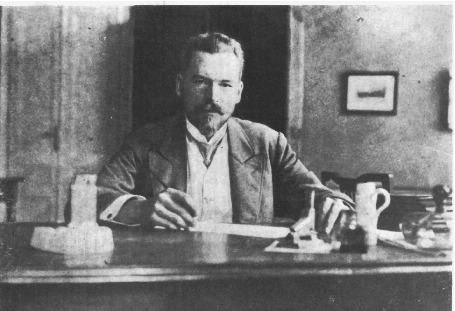#32nd M. Smoluchowski Symposium on Statistical Physics

18-21 September 2019
Kraków
Europe/Warsaw timezone

## $q$-Neighbor Majority-Vote Model on Complex Networks

20 Sep 2019, 12:40
20m
Kraków

Talk

### Speaker

Andrzej Krawiecki (Faculty of Physics, Warsaw University of Technology)

### Description

A $q$-neighbor majority-vote model for the opinion formation is introduced in which agents
represented by two-state spins update their opinions on the basis of the opinions of
randomly chosen subsets of $q$ their neighbors ($q$-lobbies). The agents with probability
$(1-2p)$, $0\le p\le1/2$, obey the majority-vote
rule in which the probability of the opinion flip depends only on the sign of the resultant
opinion of the $q$-lobby, and with probability $2p$ act independently and change opinion or
remain in the actual state with equal probabilities. Thus, the parameter $p$ controls the
degree of stochasticity in the model. In the model under study the
agents are located in the nodes of complex networks, e.g., Erd\"os-R\'enyi graphs or
scale-free networks, and the neighborhood of each agent consists of all agents
connected with him/her by edges, out of which the $q$-lobby is chosen randomly at each
step of the Monte Carlo simulation. This model is related to a recently introduced
$q$-neighbor Ising model [A.\ J\c{e}drzejewski et al., Phys.\ Rev.\ E 92, 052105 (2015);
A.\ Chmiel et al., Int.\ J.\ Modern Phys.\ C 29, 1850041 (2018)], with agents obeying
Metropolis opinion update rule, in which, in particular, first-order ferromagnetic transition was
reported, with the width of the hysteresis loop oscillating with $q$. In contrast, in the
$q$-neighbor majority vote model only second-order ferromagnetic transition is observed.
Theory for this transition is presented both in the mean-field approximation, valid for
large mean degrees of nodes and large $q$, and in a more elaborate pair approximation. In the
latter case the predicted location of the critical point $p_{c}$ agrees quantitatively with that
obtained from Monte Carlo simulations for various complex networks with broad range of
mean degrees of nodes and sizes of the $q$-lobby. Finite size scaling analysis shows that
in the vicinity of the critical point the magnetization shows scalin typical for the
mean-field Ising model, with the critical exponent $\beta = 1/2$, but other critical
exponents depend on the topology of the underlying complex network.

### Primary authors

Andrzej Krawiecki (Faculty of Physics, Warsaw University of Technology) Dr. Tomasz Gradowski (Faculty of Physics, Warsaw University of Technology)

### Presentation Materials

 Andrzej Krawiecki.pdf Video presentation
###### Your browser is out of date!

Update your browser to view this website correctly. Update my browser now

×